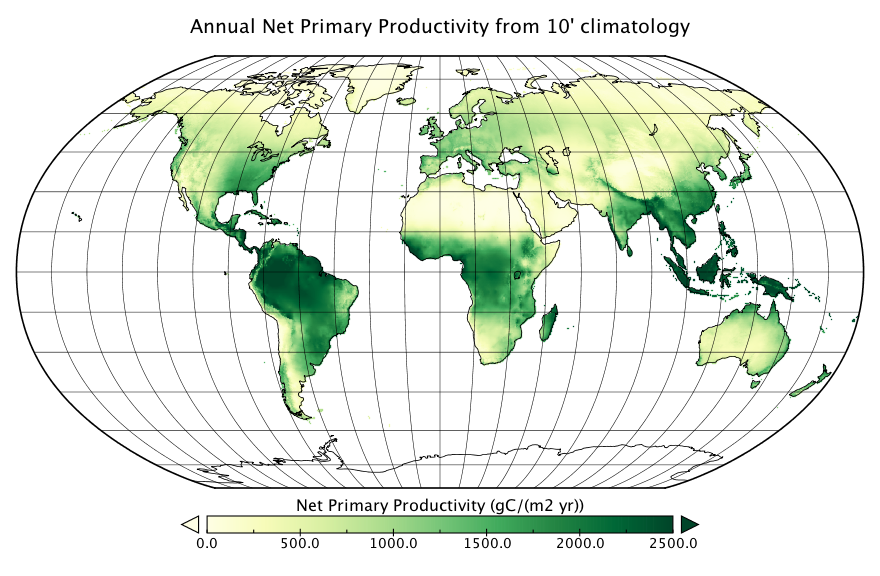I haven’t been posting for a while as I now realise. So here a new post about a fairly simple and/but old model to calculate the net primary productivity (NPP), the so called Miami Model1:

\begin{aligned} NPP(T) &= 3000/(1+\exp(1.315-0.119 T)) \\ NPP(P) &= 3000 (1-\exp(-0.000664 P)) \\ NPP &= \min(NPP(T),NPP(P)) \end{aligned}

After reading through the more recent literature, it turns out that the Miami-Model is still regarded as useful proxy for annual NPP. Global NPP observations are available, for example the Terra/MODIS Net Primary Production Yearly L4 Global 1km. But these are currently not available as global data set. Instead you can download all tiles (i.e., chunks of 1km by 1km tiles). I have no idea why, but apparently, nobody is interested in compiling it from the available tile data. Anyways, here is what the annual NPP looks like for a present-day climatology, i.e. the Ten Minute Climatology.As a Python function:

import numpy as np
def miami_model(temp,prec,a=3000.,b=1.315,c=0.119,d=0.000664):
npp_temp = a/(1.+np.exp(b-c*temp))
npp_prec = a*(1.-np.exp(-d*prec))
return np.fmin(npp_temp,npp_prec)


1. Lieth, H. (1973), Primary production: Terrestrial ecosystems, Human Ecol., 1, 303–332. link